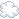# Have you ever wondered how does look the computation of Fibonnaci number in assembly?

Have you ever wondered how does look the computation of Fibonnaci number in assembly?

For sake of curiosity I chose Pep/8 Assembler and Simulator which could be found on http://code.google.com

The original C uncached version looks as following:

``````
#include <iostream>
using namespace std;
int fib (int n)
{
if (n == 0)
{
return 0;
}
else if (n == 1)
{
return 1;
}
else
{
return fib (n - 1) + fib (n - 2);
}
}
int main ()
{
int num;
cout << "Which Fibonacci number? ";
cin >> num;
cout << "The number is " << fib (num) << endl;
return 0;
}
``````

The Pep/8 implementation: